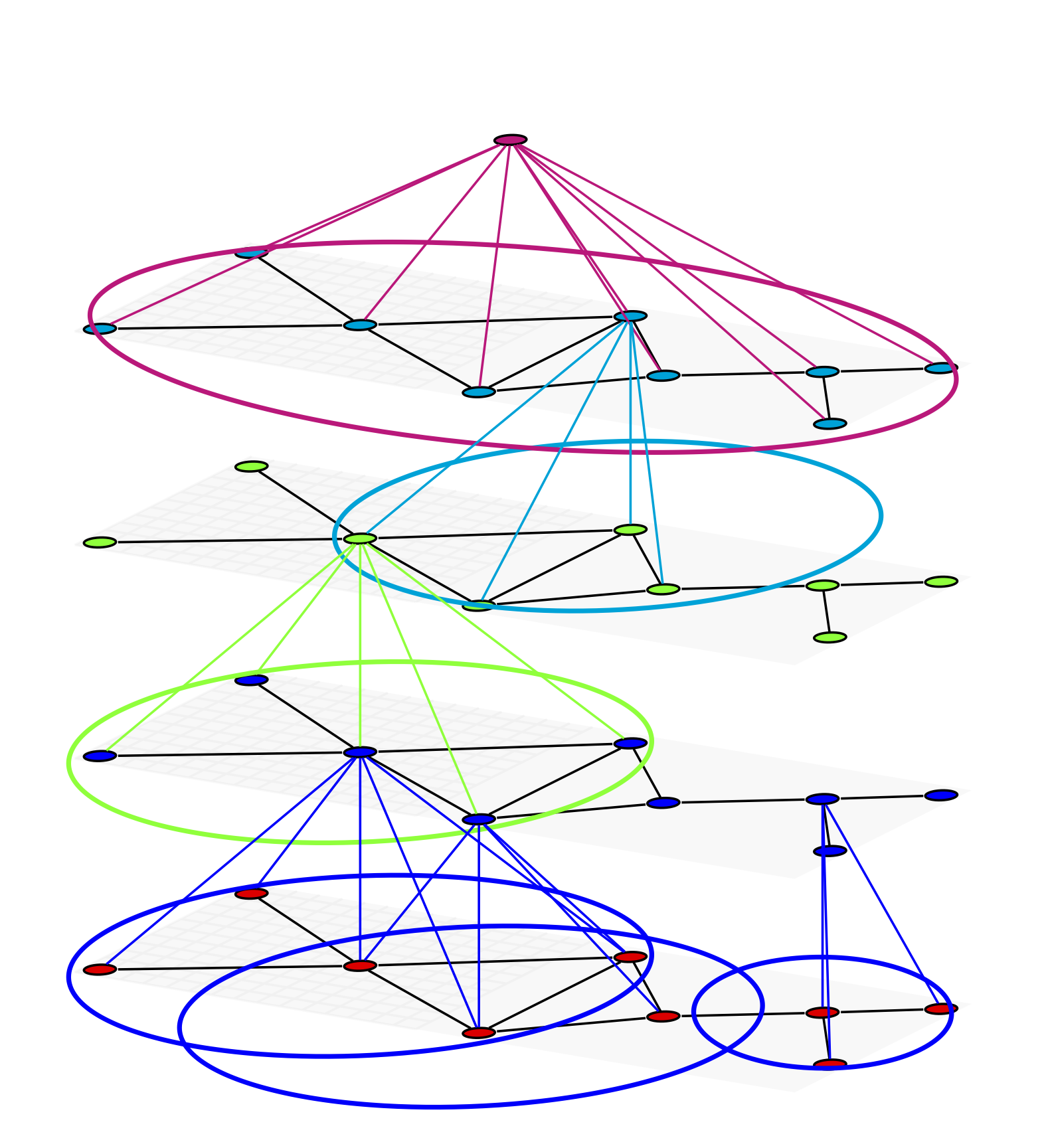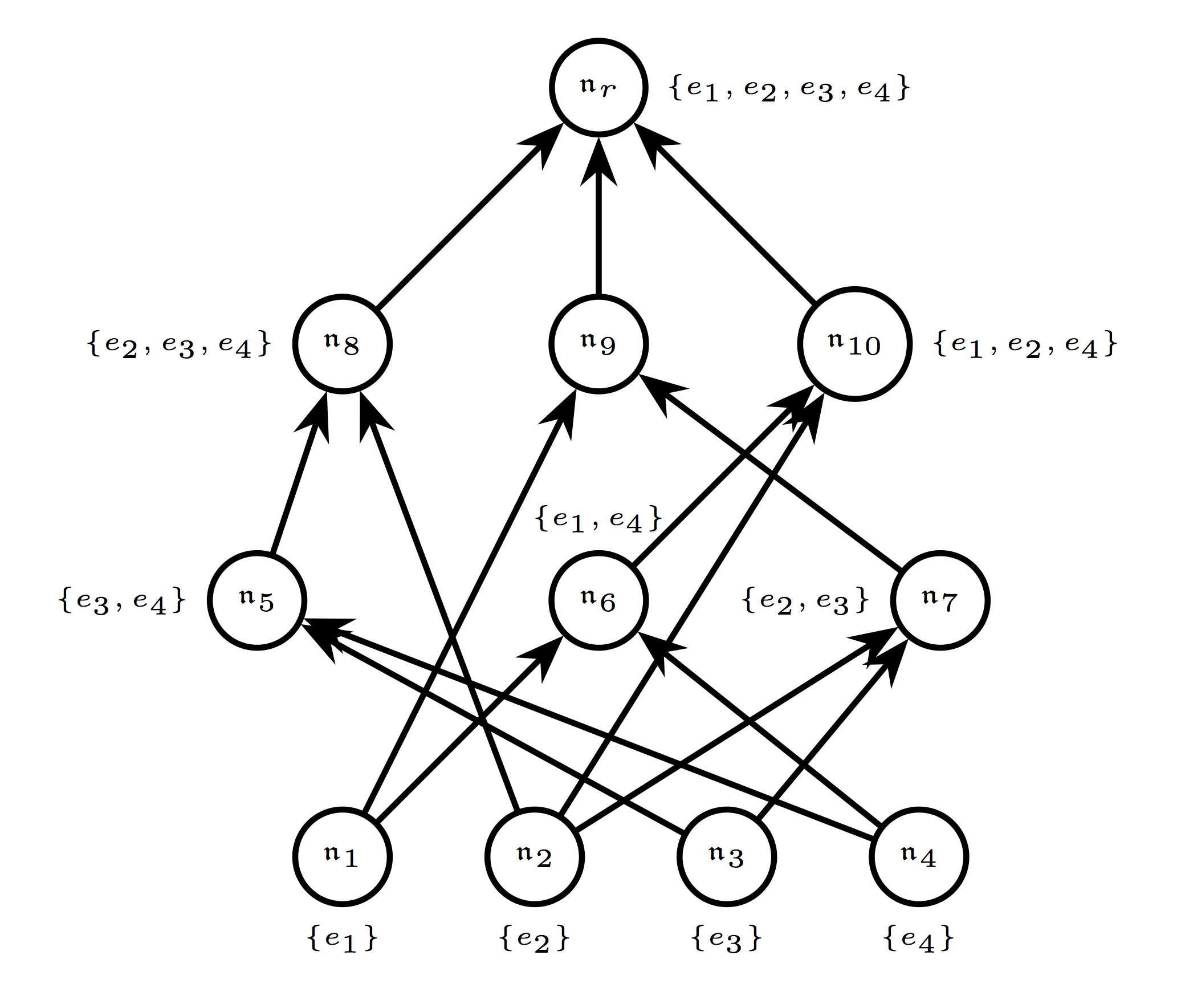# Generalized covariant neural networksIt is widely recognized that the key to phenomenal success of Convolutional Neural Networks (CNNs) is thay satisfy equivariance (more generally, covariance), i.e., that under transformations of the inputs, the activations of higher layers transform in a predictable manner (Cohen & Welling, 2016).

A number of authors have recently proposed generalizations of CNNs to combinatorial objects, specifically graphs. Many of these algorithms take the form of Message Passing Neural Networks (Gilmer et al., 2017), which aggregate` messages at each node of the graph by summation. The summation ensures permutation invariance but leads to a loss of information about the identities of vertices. In [P1] we propose a new architecture, called Covariant Compositional Neural Networks (CCNs), which are covariant to permutations rather than invariant, and thus afford a richer representation of graphs. The construction applies not only to graphs but to a wide range of structured objects with hierarchical "is-a-part-of" relationships. Implementing CCNs in an efficient manner required writing our own deep learning library called GraphFlow [S1].

One of the most important applications of CCNs is modeling molecules and atomic environments for the purpose of learning force fields (see here). This involves not just respecting permutation invariance, but also invariance to spatial transformations. In [P3] we propose N-body Networks, a fully SO(3)-covariant neural network for learning physical interactions.

On the theoretical side, in [S2] we investiagate the connection between equivariance and convolution and prove, using tools from Noncommutative Fourier Analysis, that under very general conditions, convolutional structure is a sufficient as well as necessary condition for equivariance, not just for translations, but to the action of any compact group.

### Papers

[P1]  Risi Kondor, Hy Truong Son, Horace Pan, Brandon Anderson, Shubhendu Trivedi:  Covariant compositional networks for learning graphs [arXiv 1/17/18] [video]

[P2]  Risi Kondor and Shubhendu Trivedi:  On the generalization of equivariance and convolution in neural networks to the action of compact groups [arXiv 2/11/18]

[P3]  Risi Kondor:  N-body networks: a covariant hierarchical neural network architecture for learning atomic potentials [arXiv 3/5/18]

### Software

[S1]  Hy Truong Son:  GraphFlow:  a C++ deep learning library with support for covariant compositional architectures [GitHub]